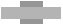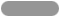# Wire Facts: Wire Length

## Round Wireft/lb = .1061 ÷ ρ x D2

Where ρ = alloy density in lb/in3, and D = wire diameter in inches.

m/kg = 1273.24 ÷ ρ x D2

Where ρ = alloy density in gm/cm3, and D = wire diameter in mm.

## Square or Rectangular Wireft/lb = .0833 ÷ ρ (w x t – .8584R2)

Where ρ = alloy density in lb/in3, w = wire length in inches, t = wire thickness in inches, and R = corner radius in inches.

m/kg = 1000 ÷ ρ (w x t – .8584R2)

Where ρ = alloy density in gm/cm3, w = wire length in mm, t = wire thickness in mm, and R = corner radius in mm.

## Round Edge Flat Wireft/lb = .0833 ÷ ρ x w x t x Cf

Where ρ = alloy density in lb/in3, w = wire width in inches, t = wire thickness in inches, and Cf = correction factor.

m/kg = 1000 ÷ ρ x w x t x Cf

Where ρ = alloy density in gm/cm3, w = wire width in mm, t = wire thickness in mm, and Cf = correction factor.

## Correction Factor (Cf)

The following chart lists the correction factor (Cf) for the area loss from a true rectangle to the round edge.

 WIDTH/THICKNESS RATIO CORRECTION FACTOR 1 .785 1.05 .805 1.1 .820 1.15 .840 1.2 .850 1.3 .865 1.4 .875 1.5 .885 1.6 .895 1.7 .905 1.8 .910 1.9 .915 2.0 .920 2.1 .925

 WIDTH/THICKNESS RATIO CORRECTION FACTOR 2.2 .930 2.3-2.4 .935 2.5-2.6 .940 2.7 .945 2.8-3.0 .950 3.1-3.3 .955 3.4-3.7 .960 3.8-4.3 .965 4.4-5.2 .970 5.3-6.2 .975 6.3-7.9 .980 8.0-9.1 .985 9.2-9.9 .990